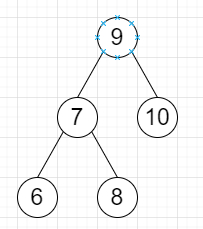# Program to check whether a binary tree is complete or not in Python

Suppose we have a binary tree; we have to check whether this is a complete binary tree or not. As we know in a complete binary tree the levels are filled with nodes except possibly the last and all nodes in the last level are as far left as possible.

So, if the input is likethen the output will be True

To solve this, we will follow these steps−

• q := a double ended queue

• insert root at the end of q

• flag := False

• while q is not empty, do

• temp := element after deleting from left of q

• if temp is null, then

• flag := True

• otherwise when flag is set and temp is not null, then

• return False

• otherwise,

• insert left of temp at the end of q

• insert right of temp at the end of q

• return True

Let us see the following implementation to get better understanding −

## Example

Live Demo

from collections import deque
class TreeNode:
def __init__(self, data, left = None, right = None):
self.data = data
self.left = left
self.right = right
class Solution:
def solve(self, root):
q = deque()
q.append(root)
flag = False
while q:
temp = q.popleft()
if not temp:
flag = True
elif flag and temp:
return False
else:
q.append(temp.left)
q.append(temp.right)
return True
ob = Solution()
root = TreeNode(9)
root.left = TreeNode(7)
root.right = TreeNode(10)
root.left.left = TreeNode(6)
root.left.right = TreeNode(8)
print(ob.solve(root))

## Input

root = TreeNode(9) root.left = TreeNode(7) root.right = TreeNode(10) root.left.left = TreeNode(6) root.left.right = TreeNode(8)

## Output

True